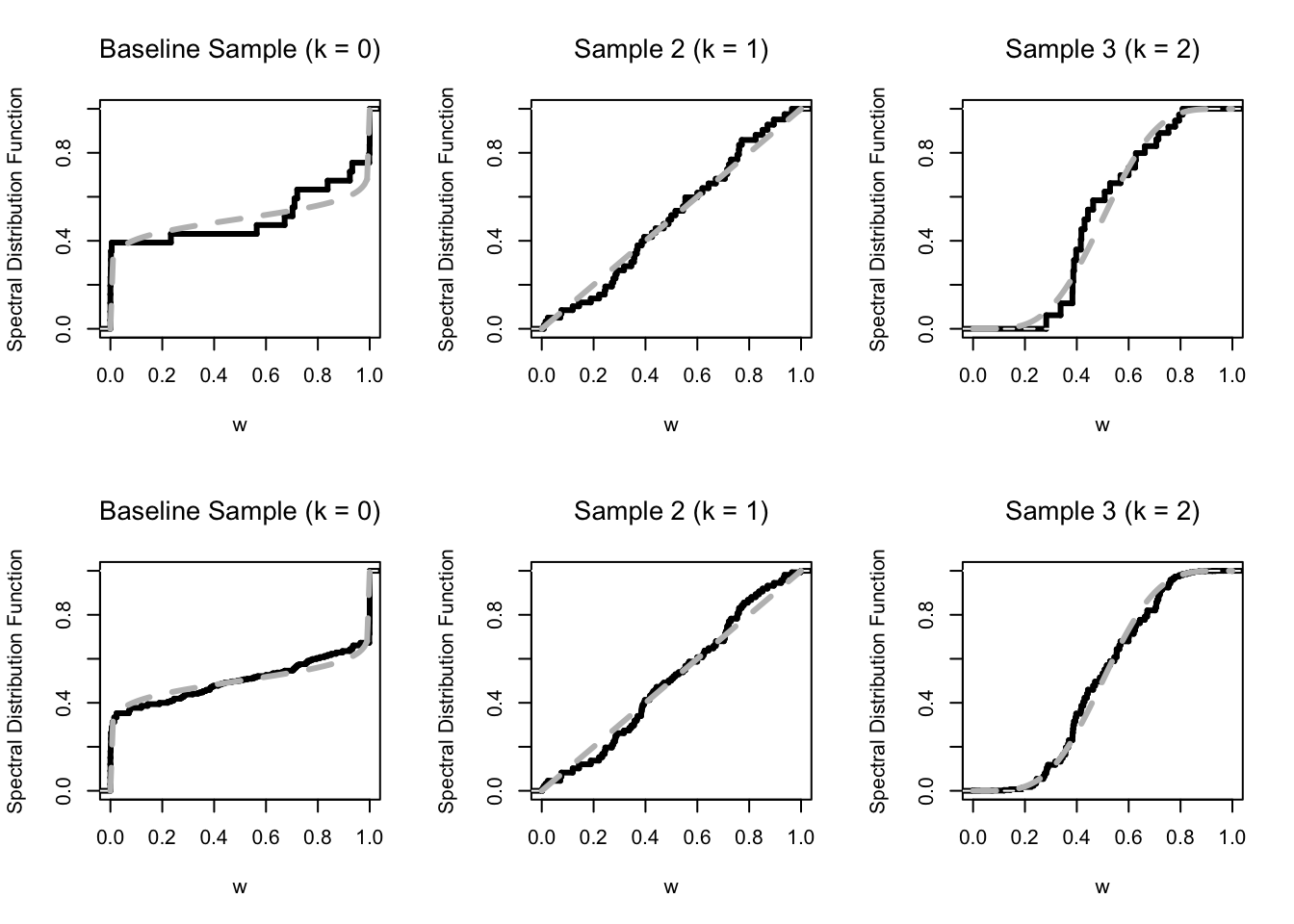Here are some notes illustrating how to fit the spectral density ratio model in de Carvalho and Davison (2014). The methods can be implemented with the alabama package or with a heuristic two-step approach described in Section 3.2 of the paper. The file aux.R includes auxiliary routines which I will be invoking below, such as simulation from the beta-spectral density ratio model (using rBeta), the log-likelihood function (LL), etc. Some notation in the codes may not match that used in the final version of the paper.

rm(list = ls())
require(emplik); require(nnet); gamma <- 0
source("aux.R") 

The goal of the example below will be on illustrating the spectral density ratio model and to compare it with the empirical likelihood spectral measure Einmahl and Segers (2009); the data are simulated according to the following settings:

n0 <- 25      ; N <- c(50, 25)     ; n <- n0 + sum(N)
rho <- N / n0 ; K <- length(N) + 1 ; K1 <- length(N)
D <- 2
## set true values of the tilt parameters
a0 <- 0.1             ; a <- c(1, 5)
## fix seed for reproducibility
set.seed(1)

We simulate the data using the routine from , and use the distortion function $$c(w) = \log\{w (1 - w)\}$$:

v <- z <- rBeta(c(n0, N), c(a0, a))
V <- log(v  * (1 - v))
Y <- c(rep(0, n0), rep(1, N),rep(2, N))

The heuristic two-stage approach can be executed using:

## nested approach: outer
fit <- multinom(Y ~ V)
## # weights:  9 (4 variable)
## initial  value 109.861229
## iter  10 value 74.784268
## final  value 72.762709
## converged
gamma0 <- c(coefficients(fit)[1, 1] + log(n0 / N),
coefficients(fit)[2, 1] + log(n0 / N),
coefficients(fit)[1, 2], coefficients(fit)[2, 2])
## nested approach: inner
delta0 <- inner(v, gamma0)

Simulation results in the paper suggest that this heuristic provides a good approximation of the tilting parameters. Yet, such inferences do not obey the normalization and moment constraints:

check.constraints(v, gamma0, delta0)
## $normalization ##  1.0196937 0.9917950 0.9967164 ## ##$moments
##  0.5098469 0.4958975 0.4983582

Full optimization can be obtained using the alabama package; the auglag routine may yield some warnings. These are due to the fact that the algorithm attempts to search outside the domain of the objective function, and thus can be safely ignored.

require(alabama)
param <- try(auglag(par = c(gamma0, delta0), fn = LL,
heq = constraints)$par) # if(is.na(param) == TRUE) # param <- try(auglag(par = c(gamma0,rep(0, K)), fn = LL, # heq = constraints)$par, silent = TRUE)

alpha <- as.numeric(param[1:(K - 1)])
beta  <- as.numeric(param[K:(2 * (K - 1))])
gamma <- as.numeric(c(alpha, beta))
delta <- as.numeric(param[(2 * (K - 1) + 1):(2 * (K - 1) + K)])

The inferences arising from the full optimization would obey moment constraints:

check.constraints(v, c(alpha, beta), delta)
## $normalization ##  1 1 1 ## ##$moments
##  0.5 0.5 0.5

To compare with the maximum empirical likelihood estimator, we compute the Lagrange multipliers:

delta0 <- el.test(v[1:n0], 1 / 2)$lambda delta1 <- el.test(v[(n0 + 1):(n0 + N)], 1 / 2)$lambda
delta2 <- el.test(v[(n0 + N + 1):n], 1 / 2)\$lambda

The results are summarized in Figure 1. On a final note, an alternative approach has been recently proposed by Castro and de Carvalho (2017).Fig. 1: Top: Maximum empirical likelihood spectral measures; Bottom: Spectral density ratio spectral measures.